ConicBundle
ConicBundle::UnconstrainedGroundset Class Reference

implements an unconstrained groundset More...

#include <UnconstrainedGroundset.hxx>

Inheritance diagram for ConicBundle::UnconstrainedGroundset: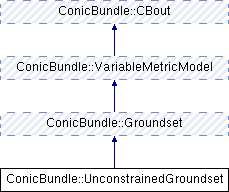## Public Member Functions

virtual void clear (CH_Matrix_Classes::Integer indim=0, CH_Matrix_Classes::Integer in_groundset_id=0)
reset everything to initial state for an unconstrained ground set of dimension indim More...

UnconstrainedGroundset (CH_Matrix_Classes::Integer indim=0, const CH_Matrix_Classes::Matrix *start_val=0, const CH_Matrix_Classes::Matrix *costs=0, const CH_Matrix_Classes::Real offset=0., CH_Matrix_Classes::Integer in_groundset_id=0)
calls clear() with the same parameters

CH_Matrix_Classes::Integer get_groundset_id () const
returns the current groundset_id, increased values indicate changes in the ground set

void set_groundset_id (CH_Matrix_Classes::Integer gsid)
sets the groundset_id to the desired value, increasing it is safer here because this is used to indicate changes

virtual CH_Matrix_Classes::Integer get_dim () const
returns the dimension of the ground set, i.e., the length of the variables vector y

virtual bool constrained () const
returns false if the feasible set is the entire space (unconstrained optimization), true otherwise. More...

virtual bool is_feasible (CH_Matrix_Classes::Integer &in_groundset_id, const CH_Matrix_Classes::Matrix &y, CH_Matrix_Classes::Real relprec=1e-10)
on input value in_groundset_id the input y was feasible. Return true if the id did not change, otherwise check if y is still feasible for the given precision. More...

virtual int ensure_feasibility (CH_Matrix_Classes::Integer &in_groundset_id, CH_Matrix_Classes::Matrix &y, bool &ychanged, BundleProxObject *Hp=0, CH_Matrix_Classes::Real relprec=1e-10)
if the groundset_id changed, it checks feasibility of y with respect to the given precision. If infeasible it replaces y by its projection with respect to the norm of Hp and sets ychanged to true. More...

virtual QPSolverObjectget_qp_solver (bool &solves_model_without_gs, BundleProxObject *Hp)
returns a pointer to an internal QPSolverObject that is able to solve bundle suproblems efficiently for this kind of groundset and scaling; if solves_model_without_gs == true the qp solver does not include the groundset and the groundset has to be dealt with by the Gauss Seidel approach

int set_qp_solver_parameters (QPSolverParametersObject *)
set parameters for the QP_Solver

virtual const CH_Matrix_Classes::Matrixget_starting_point () const
returns a stored starting point, note: this need not be feasible; if generated automatically, its dimension is correct.

virtual int set_starting_point (const CH_Matrix_Classes::Matrix &vec)
stores the a new starting point irrespective of whether it is feasible or not and returns 0 if it feasible, 1 if it is infeasible

virtual int candidate (CH_Matrix_Classes::Integer &gs_id, CH_Matrix_Classes::Matrix &newy, CH_Matrix_Classes::Real &cand_gs_val, CH_Matrix_Classes::Real &linval, CH_Matrix_Classes::Real &augval_lb, CH_Matrix_Classes::Real &augval_ub, CH_Matrix_Classes::Real &subgnorm2, const CH_Matrix_Classes::Matrix &center_y, CH_Matrix_Classes::Real center_value, const MinorantPointer &model_minorant, BundleProxObject *Hp, MinorantPointer *delta_groundset_minorant=0, CH_Matrix_Classes::Indexmatrix *delta_index=0, CH_Matrix_Classes::Real relprec=1e-2)
for a given model aggregate compute the groundset aggregate and the resulting (feasible) candidate More...

virtual const MinorantPointerget_gs_aggregate () const
returns the groundset aggregate computed in candidate()

virtual const MinorantPointerget_gs_minorant () const
returns the linear minorant valid on the entire ground set (e.g. a linear cost funciton)

virtual const CH_Matrix_Classes::Indexmatrixget_yfixed () const
if not NULL (iff get_use_yfixing()==false) it returns the vector yfixed with yfixed(i)=0 if not fixed, =1 is fixed already, =2 if newly fixed

virtual CH_Matrix_Classes::Indexmatrixset_yfixed ()
if not NULL (iff get_use_yfixing()==false) returns the vector yfixed with yfixed(i)=0 if not fixed, =1 is fixed already, =2 if newly fixed

bool get_use_yfixing () const
true if the cooridinate fixing heuristic is switched on (only constrained cases)

void set_use_yfixing (bool uyf)
set to true to switch on the cooridinate fixing heuristic (only constrained cases)

GroundsetModificationstart_modification ()
return a new modification object on the heap that is initialized for modification of *this

int apply_modification (const GroundsetModification &mdf)
change the groundset description as specified by the argument

int mfile_data (std::ostream &out) const
m-file output routine for debugging or testing in Matlab (not yet working)

void set_cbout (const CBout *cb, int incr=-1)
output settingsPublic Member Functions inherited from ConicBundle::VariableMetricModel
VariableMetricModel (CBout *cb=0, int cbincr=-1)
constructor for passing on ouptut information

virtual ~VariableMetricModel ()
virtual destructor

virtual int add_variable_metric (VariableMetric &H, CH_Matrix_Classes::Integer y_id, const CH_Matrix_Classes::Matrix &y, bool descent_step, CH_Matrix_Classes::Real weightu, CH_Matrix_Classes::Real model_maxviol, const CH_Matrix_Classes::Indexmatrix *indices=0)
add to the variable metric information H some model dependent "second order" information of the function modelled More...

virtual VariableMetricModelvariable_metric_transform ()
Overload this in order apply transformations in between. More...Public Member Functions inherited from ConicBundle::CBout
virtual void set_out (std::ostream *out=0, int print_level=1)
Specifies the output level (out==NULL: no output at all, out!=NULL and level=0: errors and warnings, level>0 increasingly detailed information) More...

void clear_cbout ()
reset to default settings (out=0,print_level=1)

CBout (const CBout *cb=0, int incr=-1)
calls set_cbout

CBout (std::ostream *outp, int pl=1)
initialize correspondingly

CBout (const CBout &cb, int incr=0)
copy constructor

virtual bool cb_out (int pl=-1) const
Returns true if out!=0 and (pl<print_level), pl<0 should be used for WARNINGS and ERRORS only, pl==0 for usual output.

std::ostream & get_out () const
If cb_out() returned true, this returns the output stream, but it will abort if called with out==0.

std::ostream * get_out_ptr () const
returns the pointer to the output stream

int get_print_level () const
returns the print_level

## Protected Attributes

CH_Matrix_Classes::Integer dim
current dimension of the ground set

CH_Matrix_Classes::Integer groundset_id
nonnegative update counter for recognizing changes in the groundset description

CH_Matrix_Classes::Matrix starting_point
the starting point

MinorantPointer gs_aggregate
the ground set aggregate, here giving the linear coefficients of the affine cost function

bool use_yfixing
if set to true in constrained versions, y-variables that are at strongly active bounds should be fixed to this value by indicating so in yfixed

CH_Matrix_Classes::Indexmatrix yfixed
in constrained versions, when use_yfixing is true, this indicates whether a coordinate should be considered as free (0), fixed (1), or newly fixed (2)

QPSolverObjectqp_solver
the QPSolver for solving the bundle subproblem with Groundset

const BundleProxObjectHp
points to the quadratic cost matrix inside ensure_feasibility() and candidate(), otherwise ==NULL

CH_Matrix_Classes::Matrix c
linear cost term for QP-subproblems

CH_Matrix_Classes::Real gamma
constant offset for QP-subproblems

## Detailed Description

implements an unconstrained groundset

This class implements an unconstrained ground set.

## ◆ candidate()

 virtual int ConicBundle::UnconstrainedGroundset::candidate ( CH_Matrix_Classes::Integer & gs_id, CH_Matrix_Classes::Matrix & newy, CH_Matrix_Classes::Real & cand_gs_val, CH_Matrix_Classes::Real & linval, CH_Matrix_Classes::Real & augval_lb, CH_Matrix_Classes::Real & augval_ub, CH_Matrix_Classes::Real & subgnorm2, const CH_Matrix_Classes::Matrix & center_y, CH_Matrix_Classes::Real center_value, const MinorantPointer & model_minorant, BundleProxObject * Hp, MinorantPointer * delta_groundset_minorant = 0, CH_Matrix_Classes::Indexmatrix * delta_index = 0, CH_Matrix_Classes::Real relprec = 1e-2 )
virtual

for a given model aggregate compute the groundset aggregate and the resulting (feasible) candidate

Let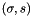be the aggregate minorant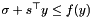of the cost function described by model_subg_offset and model_subg, let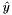be the center of stability given by center_y, let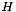denote the positive definite scaling matrix with weight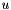given by Hp, let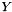denote this (convex) feasible ground set and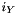its indicator function, then this computes a saddle point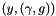of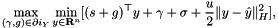The resulting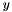is feasible and stored in newy. linval gets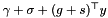, subgnorm2 gets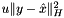, augval gets linval+subgnorm2 /2.

If delta_groundset_subg is not NULL, also elta_groundset_subg_offset and delta index are assumed to be not NULL. Then all changes from the previous groundset aggregate minorant to the new groundset aggregate minorant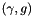are stored in delta_groundset_subg_offset and delta_groundset_subg and delta_index holds the indices of the nonzero changes (mostly the groundset aggregate is sparse, e.g. due to complementarity). This is used in BundleProxObject::update_QP_costs().

On input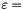relprec,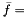center_value, and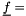augval serve to form appropriate stopping criteria if solving the saddle point problem requires a nonlinear convex optimization method. The method is then assumed to produce a primal solution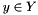of value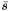and a dual solutionof value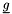with the properties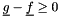and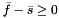and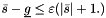. In particularis assumed to hold to machine precision.

If use_yfixing is true (the fixing heuristic is switched on), then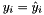is required to hold for all i with yfixed(i)!=0, so these coordinates are not allowed to change. In particular, this routine may also set yfixed(i)=2 for new coordinates i, where 2 is used to indicate newly fixed variables. These will be reset to 1 in BundlesScaling::compute_QP_costs() and BundlesScaling::update_QP_costs() when this information has been digested.

In some derived classes a scaling heuristic is called that may influence the scaling Hp so as to avoid going outside the feasible region too far.

Parameters
 [out] gs_id the current groundset_id [out] newy the next candidate y (feasible) [out] cand_gs_val the value of the groundset minorant in the candidate y (=groundset objective) [out] linval (CH_Matrix_Classes::Real&) value of linear minorant in y [in,out] augval_lb (CH_Matrix_Classes::Real&) on input: lower bound value of augmented model in previous (maybe infeasible) candidate on output: lower bound value of augmented model in y [out] augval_ub (CH_Matrix_Classes::Real&) on output: upper bound value of augmented model in y [out] subgnorm2 (CH_Matrix_Classes::Real&) squared Hp-norm (with weight) of joint groundset and model aggregate [in] center_y (const CH_Matrix_Classes::Matrix&) center of stability (feasible) [in] center_value (CH_Matrix_Classes::Real) function value in center_y [in] model_minorant (const MinorantPoiner&) aggregate linear minorant of the cost function [in,out] Hp (ConicBundle::BundleProxObject*) pointer to scaling matrix H, may be influenced by a scaling heuristic [in,out] delta_groundset_minorant (MinorantPointer*) if not NULL, the change in groundset aggregate will be stored here [in,out] delta_index (CH_Matrix_Classes::Indexmatrix*) must be not NULL iff delta_groundset_subg!=NULL or yfixed has changed, will store nonzero indices of delta_groundset_subg [in] relprec (CH_Matrix_Classes::Real) relative precision for termination in QP computations
Returns
0 on success, != 0 on failure

Implements ConicBundle::Groundset.

Referenced by set_starting_point().

## ◆ clear()

 virtual void ConicBundle::UnconstrainedGroundset::clear ( CH_Matrix_Classes::Integer indim = 0, CH_Matrix_Classes::Integer in_groundset_id = 0 )
virtual

reset everything to initial state for an unconstrained ground set of dimension indim

Note that a ground set is allowed to have dimension zero. This will lead to evaluating a function without arguments and is a realistic scenario in Lagrangean relaxation of cutting plane approaches if no cutting planes have been added yet.

If the changes to the ground set are to be counted by groundset_id, then it makes sense to enter the appropriate value in in_groundset_id.

Implements ConicBundle::Groundset.

## ◆ constrained()

 virtual bool ConicBundle::UnconstrainedGroundset::constrained ( ) const
inlinevirtual

returns false if the feasible set is the entire space (unconstrained optimization), true otherwise.

The current class implements the unconstrained case and always returns false.

Implements ConicBundle::Groundset.

References ensure_feasibility(), get_qp_solver(), and is_feasible().

## ◆ ensure_feasibility()

 virtual int ConicBundle::UnconstrainedGroundset::ensure_feasibility ( CH_Matrix_Classes::Integer & in_groundset_id, CH_Matrix_Classes::Matrix & y, bool & ychanged, BundleProxObject * Hp = 0, CH_Matrix_Classes::Real relprec = 1e-10 )
virtual

if the groundset_id changed, it checks feasibility of y with respect to the given precision. If infeasible it replaces y by its projection with respect to the norm of Hp and sets ychanged to true.

The routine is called by the internal bundle solver to check whether the given center is still valid (in some applications the groundset might change during the runtime of the bundle method), where, if ychanged is false on input, validity of y was already checked at a point in time when the groundset had the in_groundset_id. If ychanged==false and the groundset_id is still the same, then y is simply assumed to be still correct (the precision is not even looked at in this case). Otherwise the routine checks the validitiy of y with respect to the given precision. If feasible, it returns the new groundset_id in in_groundset_id and keeps ychanged unaltered. If y is infeasible, the rountine computes its projection onto the feasible set with respect to the norm of Hp (if ==0 then the Euclidean norm is used), stores it in y, sets ychanged to true, sets in_groundset_id to the current groundset_id and returns 0. Should anything go wrong, it returns 1.

This concrete base class represents the unconstrained case, so feasiblity only checks the dimension and never requires projections.

Implements ConicBundle::Groundset.

Referenced by constrained().

## ◆ is_feasible()

 virtual bool ConicBundle::UnconstrainedGroundset::is_feasible ( CH_Matrix_Classes::Integer & in_groundset_id, const CH_Matrix_Classes::Matrix & y, CH_Matrix_Classes::Real relprec = 1e-10 )
virtual

on input value in_groundset_id the input y was feasible. Return true if the id did not change, otherwise check if y is still feasible for the given precision.

The routine is called by the internal bundle solver to check whether the given center is still valid (in some applications the groundset might change during the runtime of the bundle method), where validity of y was already checked at a point in time when the groundset had the in_groundset_id. If the groundset_id is still the same, then y is simply assumed to be still correct (the precision is not even looked at in this case). Otherwise the routine checks the validitiy of y with respect to the given precision but does not enforce validity. It returns true if y is valid and false otherwise.

Implements ConicBundle::Groundset.

Referenced by constrained(), and set_starting_point().

The documentation for this class was generated from the following file: